## Proportion, Direct Variation, Inverse Variation, Joint Variation

This section defines what proportion, direct variation, inverse variation, and joint variation are and explains how to solve such equations.

#### Proportion

A proportion is an equation stating that two rational expressions are equal. Simple proportions can be solved by applying the cross products rule.

If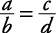, then ab = bc.

More involved proportions are solved as rational equations.

##### Example 1

Solve.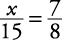Apply the cross products rule.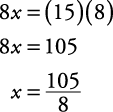The check is left to you.

##### Example 2

Solve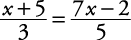.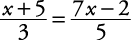Apply the cross products rule.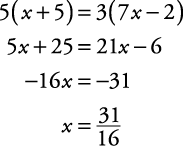The check is left to you.

##### Example 3

Solve.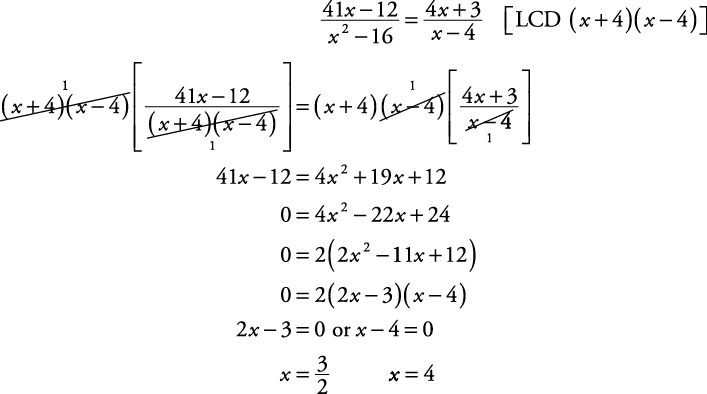However, x = 4 is an extraneous solution, because it makes the denominators of the original equation become zero. Checking to see if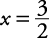is a solution is left to you.

#### Direct variation

The phrase “ y varies directly as x” or “ y is directly proportional to x” means that as x gets bigger, so does y, and as x gets smaller, so does y. That concept can be translated in two ways.

•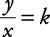for some constant k.

The k is called the constant of proportionality. This translation is used when the constant is the desired result.

•This translation is used when the desired result is either an original or new value of x or y.

• ##### Example 4

If y varies directly as x, and y = 10 when x = 7, find the constant of proportionality.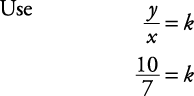The constant of proportionality is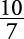.

##### Example 5

If y varies directly as x, and y = 10 when x = 7, find y when x = 12.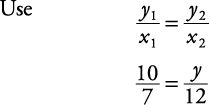Apply the cross products rule.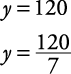#### Inverse variation

The phrase “ y varies inversely as x” or “ y is inversely proportional to x” means that as x gets bigger, y gets smaller, or vice versa. This concept is translated in two ways.

• yx = k for some constant k, called the constant of proportionality. Use this translation if the constant is desired.

• y 1 x 1 = y 2 x 2.

Use this translation if a value of x or y is desired.

##### Example 6

If y varies inversely as x, and y = 4 when x = 3, find the constant of proportionality.The constant is 12.

##### Example 7

If y varies inversely as x, and y = 9 when x = 2, find y when x = 3.#### Joint variation

If one variable varies as the product of other variables, it is called joint variation. The phrase “ y varies jointly as x and z” is translated in two ways.

•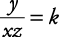if the constant is desired.

•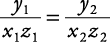if one of the variables is desired.

##### Example 8

If y varies jointly as x and z, and y = 10 when x = 4 and z = 5, find the constant of proportionality.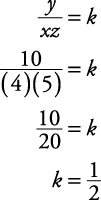##### Example 9

If y varies jointly as x and z, and y = 12 when x = 2 and z = 3, find y when x = 7 and z = 4.Occasionally, a problem involves both direct and inverse variations. Suppose that y varies directly as x and inversely as z. This involves three variables and can be translated in two ways:

•if the constant is desired.

•##### Example 10

If y varies directly as x and inversely as z, and y = 5 when x = 2 and z = 4, find y when x = 3 and z = 6.